Next: Quality of FastMatch's Up: Implementation and Performance Previous: Implementation

## Empirical Evaluation of FastMatch

Recall from Section 5 that the running time of Algorithm FastMatch is given by an expression of the form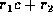. In this expression,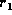represents the number of leaf node comparisons (invocations of function compare), c is the average cost of comparing leaf nodes, and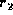represents the number of node partner checks. Partner checks are implemented in LaDiff as integer comparisons. We know thatis bounded by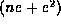, and thatis bounded by 2lne , where n is the number of tree nodes, e is the weighted edit distance between the two trees, and l is the number of internal node labels. The parameter e depends on the nature of the differences between the trees (recall the definition of weighted edit distance in Section 5.2).

There are two reasons for studying the performance of FastMatch empirically. The first reason is that the formula for the running time contains the weighted edit distance, e, which is difficult to estimate in terms of the input. A more natural measure of the input size is the number of edit operations in an optimal edit script, which we call the unweighted edit distance, d. We can show analytically that the ratio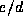is bounded by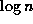for a large class of inputs, but we believe that in real cases, its value is much lower than. We therefore study the relationship between e and d empirically. The second reason is that we would like to test our conjecture that the analytical bound on the running time of FastMatch is ``loose,'' and in most practical situations the algorithm runs much faster.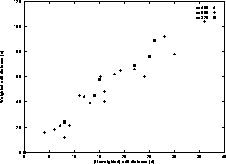Figure 11: Weighted edit distance

For our performance study, we used three sets of files. The files in each set represent different versions of a document (a conference paper). We ran FastMatch on pairs of files within each of these three sets. (Comparing files across sets is not meaningful because we would be comparing two completely different documents.) In Figure 11 we indicate how the weighted edit distance (e) varies with the unweighted edit distance (d), for each of the three document sets. Recall that n is the number of tree leaves, that is, the number of sentences in the document. We see that the relationship between e and d is close to linear. Furthermore, the variance with respect to the three document sets is not high, suggesting thatis not very sensitive to the size of the documents (n). The average value ofis 3.4 for these documents.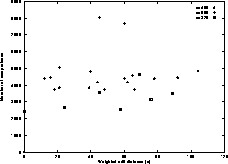Figure 12: Running time

In Figure 12 we plot how the running time of FastMatch varies with the weighted edit distance e. The vertical axis is the running time as measured by the number of comparisons made by FastMatch and the horizontal axis is the weighted edit distance. Note that the analytical bound on the number of comparisons made by FastMatch is much higher than the numbers depicted in Figure 12; on the average, FastMatch makes approximately 20 times fewer comparisons than those predicted by the analytical bound, supporting our conjecture that the analytical bound on the running time is a loose one. We also observe that Figure 12 suggests an approximately linear relation between the running time and e, although there is a high variance. This variance may be explained by our first observation that the actual running time is far below the predicted bound.Next: Quality of FastMatch's Up: Implementation and Performance Previous: Implementation

Sudarshan S. Chawathe
Wed Jun 19 08:22:34 PDT 1996